## Qualitative Analysis of ODEs

Version 1.2.0.0 (4.69 MB) by
Interactive courseware module that addresses the qualitative analysis of ordinary differential equations taught in mathematics courses.

Updated 17 Nov 2023

From GitHub

# Qualitative Analysis of ODEs

Curriculum Module

Created with R2021a. Compatible with R2021a and later releases.

# Information

This curriculum module contains interactive MATLAB® live scripts that teaches qualitative analysis of ordinary differential equations (ODEs).

## Background

You can use these live scripts as demonstrations in lectures, class activities, or interactive assignments outside class. The first two scripts consider first order ODEs. In these scripts, students draw solutions on a slope field, identify and classify equilibria, and draw phase line portraits. In the second half of the module, students analyze systems of two autonomous ODEs. In this part, students create phase plane portraits, solve for equilibria, and classify equilibria of linear systems by examining the eigenvalues of the coefficient matrix. Throughout the module, students apply the mathematical tools to study an energy balance climate model and a mass-spring-damper model.

The instructions inside the live scripts will guide you through the exercises and activities. Get started with each live script by running it one section at a time. To stop running the script or a section midway (for example, when an animation is in progress), use the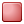Stop button in the RUN section of the Live Editor tab in the MATLAB Toolstrip.

Solutions are available upon instructor request. Contact the MathWorks teaching resources team if you would like to request solutions, provide feedback, or if you have a question.

## Prerequisites

This module assumes knowledge of differential calculus concepts and notation: both <math-renderer class="js-inline-math" style="display: inline" data-static-url="https://github.githubassets.com/static" data-run-id="154668a5f9352ca38e3e471ae8e856a6">$y^{\prime }$</math-renderer> and <math-renderer class="js-inline-math" style="display: inline" data-static-url="https://github.githubassets.com/static" data-run-id="154668a5f9352ca38e3e471ae8e856a6">$\frac{dy}{dt}$</math-renderer> are used. There is minimal MATLAB knowledge required for these scripts, but you could use MATLAB Onramp as a resource to acquire familiarity with live scripts and MATLAB syntax.

## Getting Started

### On MATLAB Online:

Use thelink to download the module. You will be prompted to log in or create a MathWorks account. The project will be loaded, and you will see an app with several navigation options to get you started.

### On Desktop:

Download or clone this repository. Open MATLAB, navigate to the folder containing these scripts and double-click on ODE_Analysis.prj. It will add the appropriate files to your MATLAB path and open an app that asks you where you would like to start.

Ensure you have all the required products (listed below) installed. If you need to include a product, add it using the Add-On Explorer. To install an add-on, go to the Home tab and select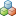Add-Ons > Get Add-Ons.

## Products

MATLAB® and Symbolic Math Toolbox™ are used throughout.

# Scripts

## SlopeFields.mlx

In this script, students will... Applications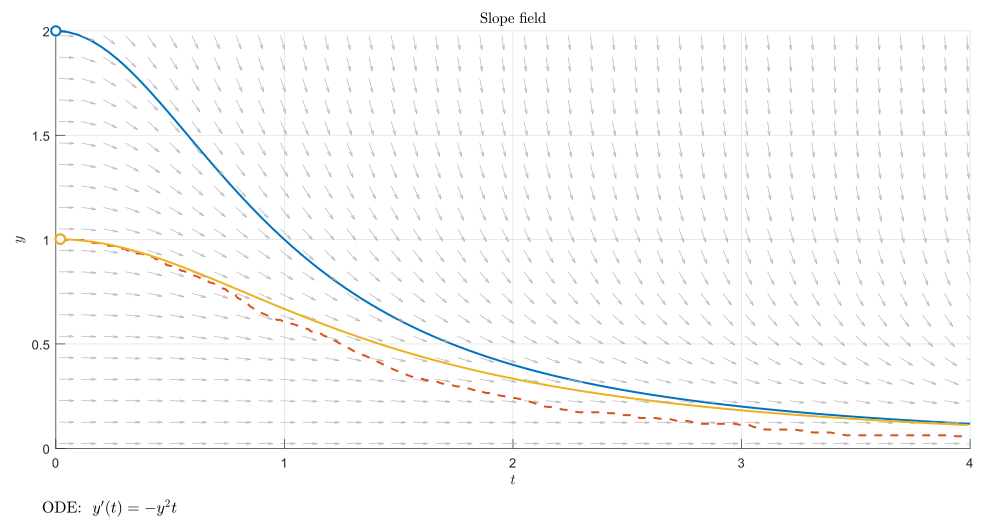- classify ODEs as autonomous or non-autonomous
- describe how a slope field is generated
- draw ODE solutions through the slope field by hand
- explain the physical meaning of terms in an energy balance climate model
- plot solutions of the energy balance climate model
Energy balance model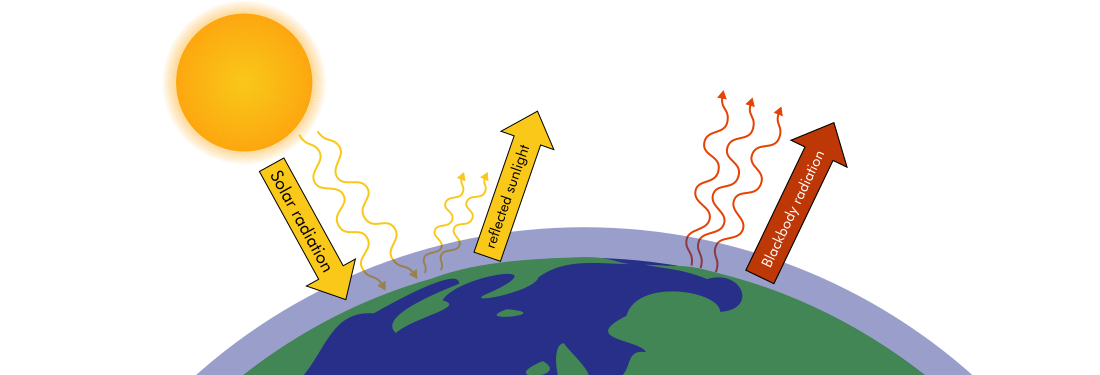## Equilibria1D.mlx

In this script, students will... Application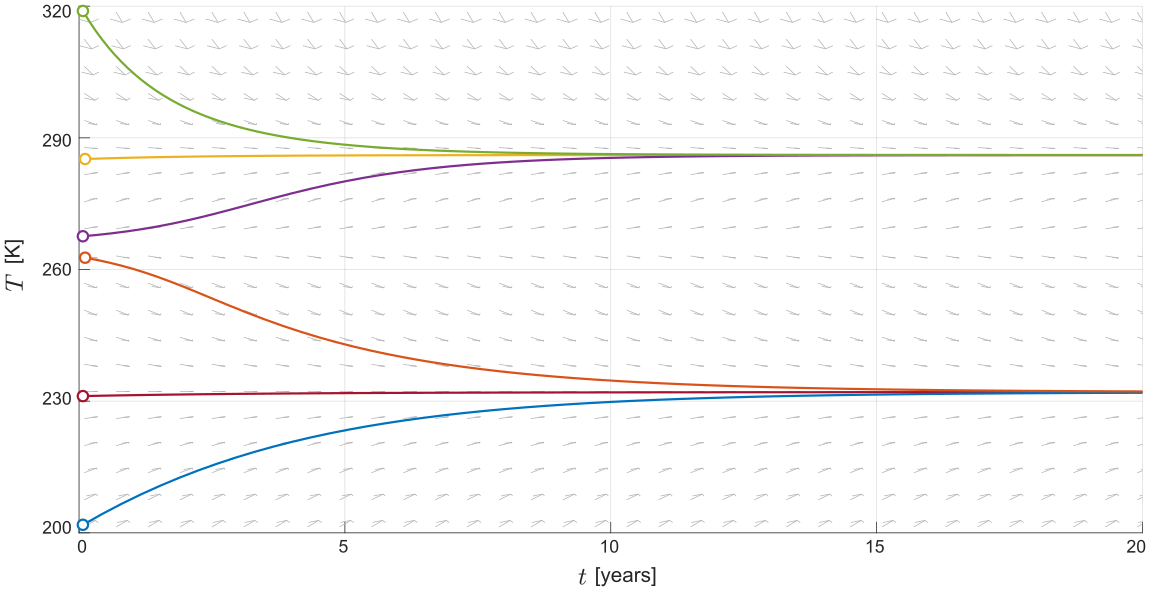- solve for equilibria of autonomous first order ODEs
- classify equilibria as stable, unstable, or semi-stable
- draw phase line portraits of autonomous ODEs and relate them to the slope field
- find the equilibria of the energy balance climate model
- describe the real-world meaning of the climate model equilibria
Energy balance model## PhasePlanes.mlx

In this script, students will... Application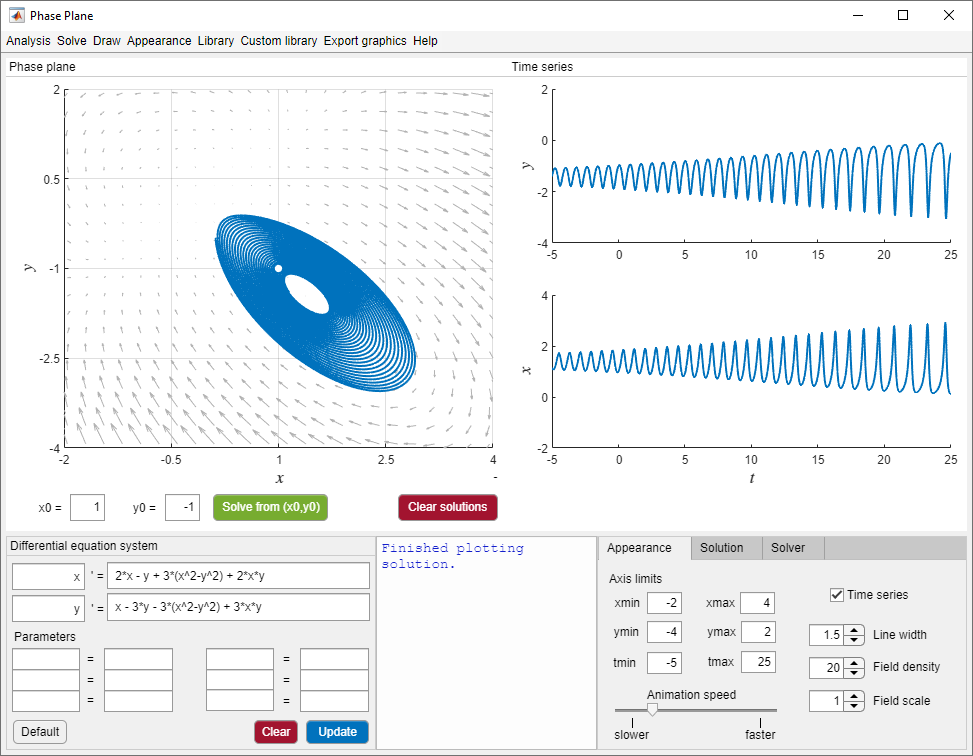- write a second order ODE as a first order ODE system
- describe how a phase plane is generated
- relate solutions plotted on the phase plane to time series solution plots
- compare behaviors of the mass-spring-damper model under variations in the damping coefficient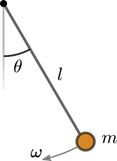## LinearPhasePlaneAnalysis.mlx

In this script, students will... Application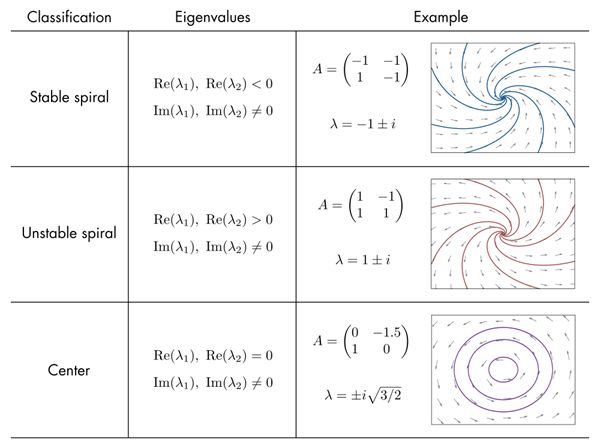- find equilibria of a second order ODE system
- compute the eigenvalues of the coefficient matrix of a linear ODE system
- classify equilibria as nodes, saddles, spirals, or centers
- categorize the behavior of the mass-spring-damper model by analyzing its coefficient matrix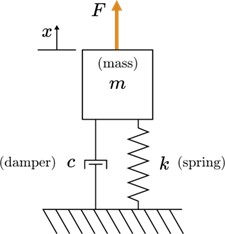# Related Courseware Modules

## Phase Plane and Slope Field

Or feel free to explore our other modular courseware content.

# Related Self-Paced Online Courses

Solving Ordinary Differential Equations with MATLAB

Learn the basics of solving ordinary differential equations in MATLAB. Use MATLAB ODE solvers to find solutions to ordinary differential equations that describe phenomena ranging from population dynamics to the evolution of the universe.

# Contribute

Looking for more? Find an issue? Have a suggestion? Please contact the MathWorks teaching resources team. If you want to contribute directly to this project, you can find information about how to do so in the CONTRIBUTING.md page on GitHub.

### Cite As

Emma Smith Zbarsky (2023). Qualitative Analysis of ODEs (https://github.com/MathWorks-Teaching-Resources/Qualitative-Analysis-of-ODEs/releases/tag/v1.2.0), GitHub. Retrieved .

##### MATLAB Release Compatibility
Created with R2021a
Compatible with R2021a and later releases
##### Platform Compatibility
Windows macOS Linux

Inspired: sinan

##### Communities
More Files in the  Distance Learning Community

### Community Treasure Hunt

Find the treasures in MATLAB Central and discover how the community can help you!

Start Hunting!

#### Utilities/OldVersions

Version Published Release Notes
1.2.0.0

See release notes for this release on GitHub: https://github.com/MathWorks-Teaching-Resources/Qualitative-Analysis-of-ODEs/releases/tag/v1.2.0

1.1.0

See release notes for this release on GitHub: https://github.com/MathWorks-Teaching-Resources/Qualitative-Analysis-of-ODEs/releases/tag/v1.1.0

1.0.0

To view or report issues in this GitHub add-on, visit the GitHub Repository.
To view or report issues in this GitHub add-on, visit the GitHub Repository.